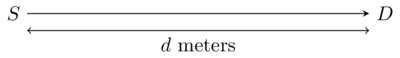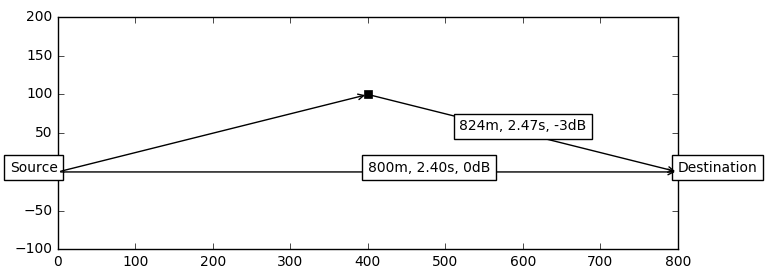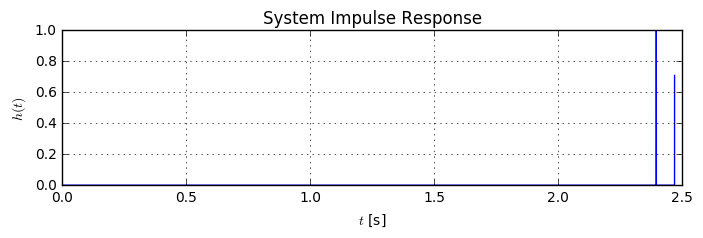# Multipath Propagation and Multipath Channel¶

In this article, we will examine the effect of multipath propagation to a transmitted signal. Furthermore, we will illustrate the effect with the help of audio examples (scroll down for the examples).

First, let's try to understand the transmission of a signal from a source $S$ to a destination $D$, which are $d$ meters apart:If we assume, the signal travels with velocity $c$, it will take $\tau=\frac{d}{c}$ seconds until it arrives at the destination. Mathematically, we have

$$y(t)=x\left(t-\frac{d}{c}\right),$$

where $x(t)$ is the transmitted signal and $y(t)$ is the received signal. Now, we know that the signal from the source is not only transmitted into the direction of $D$, but in all directions. Let's assume somewhere around the source, there is a big wall that can reflect the signal:So, at the destination we receive two copies of the signal: One over the path of $d$ meters and one over the path of $d_1$ + $d_2$ meters. Let us assume that the reflection of the signal at the wall removes 50% of the amplitude from the signal. Then, the received signal at the destination can be mathematically written as

$$y(t)=x\left(t-\frac{d}{c}\right) + 0.5\cdot x\left(t-\frac{d_1+d_2}{c}\right).$$

This characteristic is named multipath propagation, since the signal can arrive at the destination via multiple different paths. We can express the received signal as a convolution. Remember the time-shifting rule for the Dirac Delta function

$$a(t)*\delta(t-\tau) = a(t-\tau),$$

i.e. convolving a signal $a(t)$ with a time-shifted Dirac Delta amounts to a time-shift of the convolved signal $a(t-\tau)$. Using this property, we can express the received signal by

$$y(t) = x(t)*\left[\delta\left(t-\frac{d}{c}\right)+0.5\cdot\delta\left(t-\frac{d_1+d_2}{c}\right)\right].$$

If we replace $\tau_1=\frac{d}{c}$, $\tau_2=\frac{d_1+d_2}{c}$, $a_1=1$, $a_2=0.5$, we can write $$y(t)=x(t)*\left[a_1\delta(t-\tau_1)+a_2\delta(t-\tau_2)\right].$$

In general, there can be more than 2 paths for the transmission, so we can generalize this formula to $$y(t) =x(t)*\sum_{n=1}^{L}a_n\delta(t-\tau_n)=x(t)*h(t),$$ where $L$ denotes the number of paths, $a_n$ is the amplitude for this path and $\tau_n$ is the delay for this path. We can combine all multipaths into the function $h(t)$, which describes the impulse response of the system.

Hence, the system is a linear timeinvariant (LTI) system, and vast mathematical analysis can be done on these types of systems. For now, let us just listen to the effect that multipath propagation has on an audio signal. You can imagine, it sounds like an echo in the mountains.

First, let's load some audio files from the internet. We resample them to 10kHz sampling frequency to save some bandwidth and to have a common sampling rate. Here's a function which loads an audio file from the internet:

def loadAudio(url, start, length):
R = requests.get(url)
with open("sound.mp3", "wb") as f:
f.write(R.content)
!ffmpeg -y -i sound.mp3 sound.wav > /dev/null 2>&1
if len(data.shape) > 1:
data = data.sum(axis=1)
data = (1.0 * data / abs(data).max()).astype(np.float32)

dataPart = data[rate*start+np.arange(min(rate*length, len(data)))]
targetRate = 10000   # Resample signal to 10kHz sampling rate
assert (rate > targetRate)
targetSamples = int(len(dataPart) * targetRate / rate)
resampled = signal.resample(dataPart, targetSamples)
return targetRate, resampled


Below is just some convenience function to show two audio samples side by side.

def audioSideBySide(name1, audio1, name2, audio2):
text = "%s%s%s%s" % (name1, name2, audio1._repr_html_(), audio2._repr_html_())
display(HTML(text))


Now, let's load some sounds from the internet and listen to them:

url_voice = "http://ia800200.us.archive.org/21/items/adventures_of_dog_1101_librivox/adventuresofadog_00_elwes.mp3"
url_music = "http://www.scientificinvesting.eu/a/Mozart%20-%20Symphony%20n.10%20K.74%20in%20G%20-%201%20Allegro.mp3"
rate_voice, data_voice = loadAudio(url_voice, 40, 10)
rate_music, data_music = loadAudio(url_music, 40, 10)
rate_slap, data_slap = loadAudio("http://soundbible.com/grab.php?id=1948&type=mp3", 0, 2)
rate_morse, data_morse = loadAudio("http://soundbible.com/grab.php?id=1170&type=mp3", 0, 2)
rate = rate_slap

audioSideBySide("Slap", Audio(data=data_slap, rate=rate_slap), "Morse", Audio(data=data_morse, rate=rate_morse))
audioSideBySide("Music", Audio(data=data_music, rate=rate_music), "Voice", Audio(data=data_voice, rate=rate_voice))

 Slap Morse Your browser does not support the audio element. Your browser does not support the audio element.
 Music Voice Your browser does not support the audio element. Your browser does not support the audio element.

We have a short, impulse-like "slap" sound. We can use this sound to easily hear the different echos from the multipath transmission. Then, we have some signal of a morse code transmission. We will hear, that successful decoding is very hard, once there exists some multipath. Finally, we have voice and music samples to examine the effect of the multipath.

Let us define a function visualizeMultipath which calculates the effectice channel impulse response, given coordinates of source, destination and some reflectors. Additionally, it draws a diagram of the locations of the source, destination and reflectors.

c = 334  # Speed of sound in air
def visualizeMultipath(source, dest, reflectors):
tau_list = []  # list of delays
amp_list = [] # list of amplitudes
plt.plot(source, source, 'x')
plt.plot(dest, dest, 'o')
dist = np.linalg.norm(dest - source);
tau = dist / c
tau_list.append(tau); amp_list.append(0)
for r_pos, r_amp in reflectors:
plt.plot(r_pos, r_pos, 'sk')
dist = np.linalg.norm(r_pos-source) + np.linalg.norm(dest-r_pos)
tau = dist / c
tau_list.append(tau); amp_list.append(r_amp)

# Now, calculate the impulse response. Here, we have to round the echo times to the nearest integer sample time
h = np.zeros(int(rate*max(tau_list))+1)
for tau, amp in zip(tau_list, amp_list):
h[int(tau*rate)] += 10**(amp/20)
t = np.arange(len(h)) / rate
return t, h


Let us start with a setup with a single reflector. The reflectors attenuates the signal by 50% of the power, which amounts to a attenuation of $-3$dB. You can have a loot at the article about dB to linear conversion for an explanation.

reflectors = [((400,100), -3)] # One reflector at x=400, y=100 with 3dB attenuation

t, h = visualizeMultipath((0,0), (800,0), reflectors);  # source and destination are 800m apart.
plt.plot(t, h);We see two different paths from the source to the destination, where the second path has a smaller amplitude then the first one, since it attenuates the signal. Let's listen to the effect of this system to our sound samples:

audioSideBySide("Original Slap", Audio(data=data_slap, rate=rate),